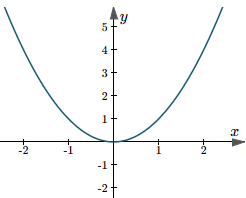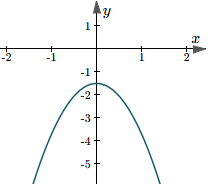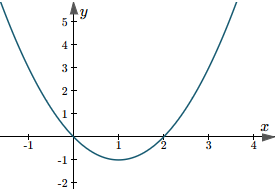Search IntMath
Close

# New applet: What does b do in a quadratic function?

By Murray Bourne, 30 Jun 2017

The parabola is one of the most common curves we come across in engineering and science, as it is often an appropriate choice for modelling portions of curves because of its simplicity.

We've seen elsewhere how to Draw the Graph of a Quadratic Equation (a parabola), and about Parabolas with Vertical and Horizontal Axes. Moving in the reverse direction, we learned how to Find the equation of a quadratic function from its graph.

In this new applet, we learn the effects of changing each of the a, b and c variables in the quadratic form of a parabloa,

y = ax2 + bx + c

## Changing a and c

Changing variables a and c are quite easy to understand, as you'll discover in the applet. You'll see something like the following as you move the sliders:## Changing b

The effect of changing variable b is not so clear. The original curve seems to move around a new curve. What is that new curve and how much does the original curve move by?## The new applet

Time to check out the applet at:

### Comment Preview

HTML: You can use simple tags like <b>, <a href="...">, etc.

To enter math, you can can either:

1. Use simple calculator-like input in the following format (surround your math in backticks, or qq on tablet or phone):
a^2 = sqrt(b^2 + c^2)
(See more on ASCIIMath syntax); or
2. Use simple LaTeX in the following format. Surround your math with $$ and $$.
$$\int g dx = \sqrt{\frac{a}{b}}$$
(This is standard simple LaTeX.)

NOTE: You can mix both types of math entry in your comment.